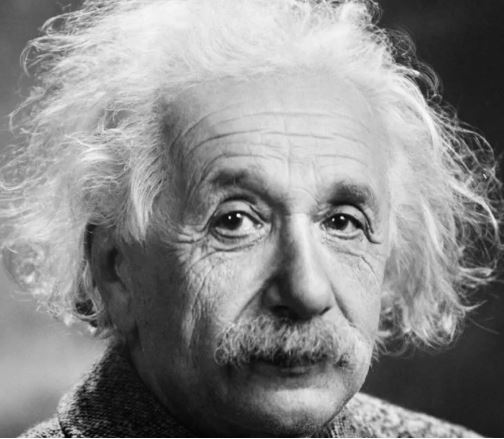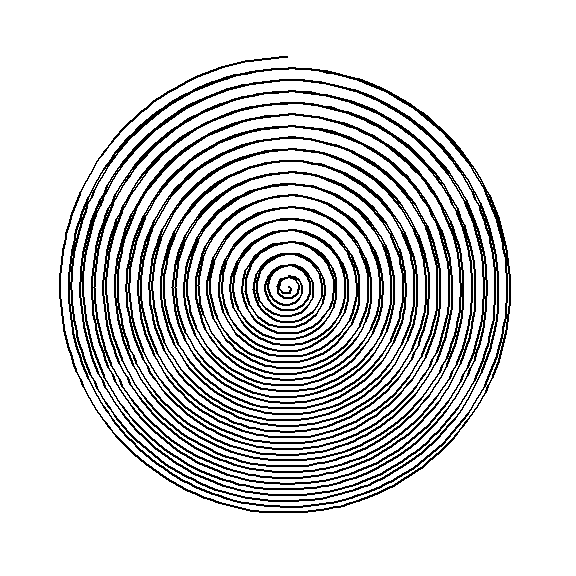# How To: Spiral line drawings with the tidyverse and gganimate

Golden Girls
R
gganimate
Convert an image into a single-line spiral drawing using R and the tidyverse.
Author

Ryan

Published

September 11, 2018

Note

Originally published on September 11, 2018. Slightly revisited and revised on August 7, 2022.

### Introduction

I stumbled on this video from Instagram of an artist creating a picture of Marilyn Monroe by drawing a single spiral from the inside out, varying the thickness of the line to add light and shadow to the image.

After watching the video on loop way too many times, I decided that I had to try and see if could do the same. Given that my drawing skills are near-zero, I turned to R and ggplot. Turns out, this was also a great opportunity to learn Thomas Pedersen’s gganimate package for turning ggplots into animated gifs.

### The Goal

Using R, the tidyverse, and gganimate, reproduce a photo as a spiral using just a single line of varying thickness.

### Prepare the image

Like the video, we’ll reproduce an iconic black-and-white image, though I chose a portrait of Albert Einstein instead.As a first step, we resize the image. I had previously written some functions to convert an image into a LEGO mosaic. We can use the `scale_image()` function from this project, which takes a 3-dimensional JPG or PNG matrix (width, height, RGB channel), crops it into a square, and converts it to a tidy data frame for plotting.

``````#1 SCALE IMAGE ----
# Adapted from LEGO mosaics project
scale_image <- function(image, img_size){
#Convert image to a data frame with RGB values
img <- bind_rows(
list(
(as.data.frame(image[, , 1]) %>%
mutate(y=row_number(), channel = "R")),
(as.data.frame(image[, , 2]) %>%
mutate(y=row_number(), channel = "G")),
(as.data.frame(image[, , 3]) %>%
mutate(y=row_number(), channel = "B"))
)
) %>%
gather(x, value, -y, -channel) %>%
mutate(x = as.numeric(gsub("V", "", x))) %>%

img_size <- round(img_size, 0)

#Wide or tall image? Shortest side should be `img_size` pixels
if(max(img\$x) > max(img\$y)){
img_scale_x <-  max(img\$x) / max(img\$y)
img_scale_y <- 1
} else {
img_scale_x <- 1
img_scale_y <-  max(img\$y) / max(img\$x)
}

#If only 1 img_size value, create a square image
if(length(img_size) == 1){
img_size2 <- c(img_size, img_size)
} else {
img_size2 <- img_size[1:2]
img_scale_x <- 1
img_scale_y <- 1
}

#Rescale the image
img2 <- img %>%
mutate(y_scaled = (y - min(y))/(max(y)-min(y))*img_size2*img_scale_y + 1,
x_scaled = (x - min(x))/(max(x)-min(x))*img_size2*img_scale_x + 1) %>%
select(-x, -y) %>%
group_by(y = ceiling(y_scaled), x = ceiling(x_scaled)) %>%
#Get average R, G, B and convert it to hexcolor
summarize_at(vars(R, G, B), funs(mean(.))) %>%
rowwise() %>%
mutate(color = rgb(R, G, B)) %>%
ungroup() %>%
#Center the image
filter(x <= median(x) + img_size2/2, x > median(x) - img_size2/2,
y <= median(y) + img_size2/2, y > median(y) - img_size2/2) %>%
#Flip y
mutate(y = (max(y) - y) + 1)

out_list <- list()
out_list[["Img_scaled"]] <- img2

return(out_list)
}``````

We will want our final spiral to have a radius of 50px (`radius`), so we can pass `radius * 2` to the scaling function, creating a 100 pixel x 100 pixel image.

``````library(tidyverse)
library(jpeg)

radius <- 50 #pixels
einstein <- readJPEG("Einstein.jpg") %>%

einstein\$Img_scaled %>%
ggplot(aes(x=x, y=y, fill=color)) +
geom_raster() +
scale_fill_identity(guide = FALSE) +
labs(title = "Scaled Einstein image",
subtitle = "100px * 100px") +
coord_fixed() +
theme_void()``````### Polar vs Cartesian coordinates

Drawing a spiral in polar coordinates is easy enough…

``````tibble(x = rep(c(1:20), 20), y = 1:400) %>%
ggplot(aes(x=x, y=y)) +
geom_path() +
coord_polar() +
theme_void()``````Using that process, I originally tried to convert the image x- and y-values into polar coordinates beginning in the center of the image. That task turned out to be much more difficult than I had imagined.

Instead, I opted to draw a spiral in Cartesian coordinates. It’s been 10 years since my last trigonometry class, but found this helpful post on Stack Overflow. Based off the first answer on the thread, I wrote a function to calculate the points of a spiral centered on the image. All points on this spiral are equidistant, so more points are on the outer sections of the spiral than the inner sections.

``````# Function for equidistant points on a spiral

spiral_cartesian <- function(img_df, spiral_radius, num_coils, chord_length, rotation){
img <- img_df\$Img_scaled

#Derive additional spiral specifications
centerX <- median(img\$x)
centerY <- median(img\$y)

thetaMax <- num_coils * 2 * pi
awayStep <- spiral_radius / thetaMax

#While loop to keep drawing spiral until we hit thetaMax
spiral <- tibble()
theta <- chord_length/awayStep

while(theta <= thetaMax){
#How far away from center
away = awayStep * theta

#How far around the center
around = theta + rotation

#Convert 'around' and 'away' to X and Y.
x = centerX + cos(around) * away
y = centerY + sin(around) * away

spiral <- spiral %>%
bind_rows(tibble(x=x, y=y))

theta = theta + chord_length/away
}

return(c(img_df, list(spiral = spiral)))
}``````

We can then pass the `einstein` image into this function (this provides the function with the desired x- and y-limits), along with specifications for the radius of the spiral (defined earlier), the number of coils, the chord length (distance between each point), and the rotation. We can plot this spiral using `coord_fixed()` - Cartesian coordinates rather than the polar coordinates above.1

``````einstein <- einstein %>%
num_coils     = 50, #Spiral folds on itself 50 times
chord_length  = 2,  #Each point is 2 pixels apart
rotation      = 0   #No rotation
)

einstein\$spiral %>%
ggplot(aes(x=x, y=y)) +
geom_path() +
coord_fixed() + #Not polar!
theme_void()``````### Mapping the image onto the spiral

The artist in the video is able to portray Marilyn Monroe by varying the thickness of the line while drawing a continuous spiral. Now that we have the our spiral in Cartesian coordinates and have scaled it to same size as the photo of Einstein, we need to vary the thickness.

We can do this by effectively overlaying the spiral on top of the image and then assigning the color of the closet image pixel(s) to the spiral point. In this function, I convert the three color channels to an inverted grey scale, where the value of 0 means white, 1 means black, and anything in between is a shade of grey.

``````#Project the image onto the spiral
project_image <- function(img_df){
dat <- img_df\$spiral %>%
#Round each spiral point to nearest whole number
mutate(xs = round(x), ys = round(y)) %>%
#Join on the rounded points
left_join(img_df\$Img_scaled %>% rename(xs=x, ys=y)) %>%
#Create greyscale - 0 is lightest, 1 is darkest
mutate(grey = R+G+B,
grey = (1- (grey / max(grey))))

return(c(img_df, list(projected_spiral = dat)))
}``````

We plot the spiral again, but this time, use the grey value to scale the thickness2 of the line. To increase the contrast of the photo, raise the grey value to a power greater than 1.

``````einstein <- einstein %>%
project_image()

einstein\$projected_spiral %>%
ggplot(aes(x=x, y=y, size = grey^(5/4))) +
geom_path() +
scale_size_continuous(range = c(0.1, 1.8),
guide = FALSE) +
coord_fixed(expand = FALSE) +
theme_void()``````…Boom! A single spiral of varying line thickness to render an image of Albert Einstein.

### Animating the spiral

The original video seems way more impressive than this image. I can’t compete with a hand-drawn image, but can add some drama to this project by animating the spiral, slowly drawing it from the inside out.

We can do this using the package gganimate, which converts ggplots into animated gifs3.

Warning

This post and writing was done with a previous version of gganimate. The tips and script here will need to be updated for the new version.

``````library(gganimate)

p <- einstein\$projected_spiral %>%
mutate(row_number = row_number()^(1/2)) %>%  #^(1/2) slows down the beginning of the drawing
ggplot(aes(x=x, y=y, size = grey)) + #Original contrast for this larger drawing
geom_path() +
scale_size_continuous(range = c(0.1, 1.8),
guide = FALSE) +
coord_fixed(expand = FALSE) +
theme_void() +
transition_reveal(row_number)``````Adding `gganimate` functions to an existing ggplot is simple. We add one new series, `row_number`, to our data frame, which is used in the final plotting step. Adding `+ transition_reveal(1, row_number)` to the ggplot instructions tells R to render this as an animation, revealing all the data as a single group (`1`) over the time span `row_number`. By using the square root of `row_number()`, `gganimate` will spend more time drawing the inner sections of the spiral, speeding up as it reaches the outer lines.

Project: ✅ Done

The spiral drawing function can be used for color images as well. This doesn’t make much sense for simulating a hand-drawn image using a marker, but still produces a fun image.

``````goldengirls <- readJPEG("GoldenGirls.jpg") %>%
scale_image(radius * 2) %>%
num_coils     = 50, #Spiral folds on itself 50 times
chord_length  = 2,  #Each point is 2 pixels apart
rotation      = 0   #No rotation
)  %>%
project_image()

goldengirls\$projected_spiral %>%
mutate(row_number = row_number()^(1/2)) %>%
ggplot(aes(x=x, y=y, size = grey,
color = color)) +
geom_path(aes(group=1)) + #Add a group to tell it to draw a single line
scale_size_continuous(range = c(0.1, 1.8),
guide = FALSE) +
scale_color_identity(guide = FALSE) +
coord_fixed(expand = FALSE) +
theme_void() +
transition_reveal(1, row_number)``````And finally, let’s end where we began, by rendering the image of Marilyn Monroe as an animated spiral.

``````marilyn <- readJPEG("Marilyn.jpg") %>%
scale_image(radius * 2) %>%
num_coils     = 50, #Spiral folds on itself 50 times
chord_length  = 2,  #Each point is 2 pixels apart
rotation      = 0   #No rotation
)  %>%
project_image()

marilyn\$projected_spiral %>%
mutate(row_number = row_number()^(1/2)) %>%
ggplot(aes(x=x, y=y, size = grey)) +
geom_path() +
scale_size_continuous(range = c(0.1, 1.8),
guide = FALSE) +
coord_fixed(expand = FALSE) +
theme_void() +
transition_reveal(1, row_number)``````Try it out! Full script can be found on GitHub!

## Footnotes

1. The plotted spiral seems to have reflections like a vinyl record. I assume that’s from the rendering of the plot at this resolution.↩︎

2. The `range` values in `scale_size_continuous()` will require a bit of trial & error and will depend on the final size of the plotted spiral.↩︎

3. If you’re using a Windows OS with R 3.5, you might have some trouble installing this package. I recommend reinstalling RTools, referring to this thread, and using this script.↩︎